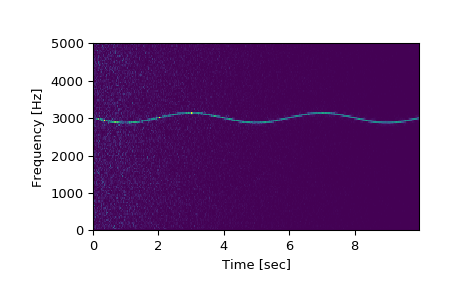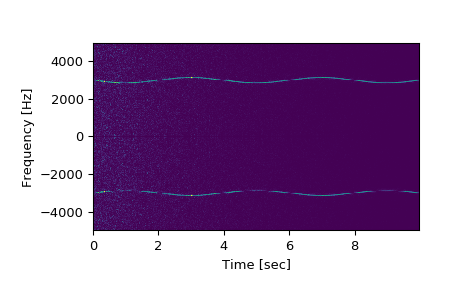# scipy.signal.spectrogram¶

scipy.signal.spectrogram(x, fs=1.0, window=('tukey', 0.25), nperseg=None, noverlap=None, nfft=None, detrend='constant', return_onesided=True, scaling='density', axis=-1, mode='psd')[source]

Compute a spectrogram with consecutive Fourier transforms.

Spectrograms can be used as a way of visualizing the change of a nonstationary signal’s frequency content over time.

Parameters: x : array_like Time series of measurement values fs : float, optional Sampling frequency of the x time series. Defaults to 1.0. window : str or tuple or array_like, optional Desired window to use. If window is a string or tuple, it is passed to get_window to generate the window values, which are DFT-even by default. See get_window for a list of windows and required parameters. If window is array_like it will be used directly as the window and its length must be nperseg. Defaults to a Tukey window with shape parameter of 0.25. nperseg : int, optional Length of each segment. Defaults to None, but if window is str or tuple, is set to 256, and if window is array_like, is set to the length of the window. noverlap : int, optional Number of points to overlap between segments. If None, noverlap = nperseg // 8. Defaults to None. nfft : int, optional Length of the FFT used, if a zero padded FFT is desired. If None, the FFT length is nperseg. Defaults to None. detrend : str or function or False, optional Specifies how to detrend each segment. If detrend is a string, it is passed as the type argument to the detrend function. If it is a function, it takes a segment and returns a detrended segment. If detrend is False, no detrending is done. Defaults to ‘constant’. return_onesided : bool, optional If True, return a one-sided spectrum for real data. If False return a two-sided spectrum. Note that for complex data, a two-sided spectrum is always returned. scaling : { ‘density’, ‘spectrum’ }, optional Selects between computing the power spectral density (‘density’) where Sxx has units of V**2/Hz and computing the power spectrum (‘spectrum’) where Sxx has units of V**2, if x is measured in V and fs is measured in Hz. Defaults to ‘density’. axis : int, optional Axis along which the spectrogram is computed; the default is over the last axis (i.e. axis=-1). mode : str, optional Defines what kind of return values are expected. Options are [‘psd’, ‘complex’, ‘magnitude’, ‘angle’, ‘phase’]. ‘complex’ is equivalent to the output of stft with no padding or boundary extension. ‘magnitude’ returns the absolute magnitude of the STFT. ‘angle’ and ‘phase’ return the complex angle of the STFT, with and without unwrapping, respectively. f : ndarray Array of sample frequencies. t : ndarray Array of segment times. Sxx : ndarray Spectrogram of x. By default, the last axis of Sxx corresponds to the segment times.

periodogram
Simple, optionally modified periodogram
lombscargle
Lomb-Scargle periodogram for unevenly sampled data
welch
Power spectral density by Welch’s method.
csd
Cross spectral density by Welch’s method.

Notes

An appropriate amount of overlap will depend on the choice of window and on your requirements. In contrast to welch’s method, where the entire data stream is averaged over, one may wish to use a smaller overlap (or perhaps none at all) when computing a spectrogram, to maintain some statistical independence between individual segments. It is for this reason that the default window is a Tukey window with 1/8th of a window’s length overlap at each end.

New in version 0.16.0.

References

  Oppenheim, Alan V., Ronald W. Schafer, John R. Buck “Discrete-Time Signal Processing”, Prentice Hall, 1999.

Examples

>>> from scipy import signal
>>> import matplotlib.pyplot as plt


Generate a test signal, a 2 Vrms sine wave whose frequency is slowly modulated around 3kHz, corrupted by white noise of exponentially decreasing magnitude sampled at 10 kHz.

>>> fs = 10e3
>>> N = 1e5
>>> amp = 2 * np.sqrt(2)
>>> noise_power = 0.01 * fs / 2
>>> time = np.arange(N) / float(fs)
>>> mod = 500*np.cos(2*np.pi*0.25*time)
>>> carrier = amp * np.sin(2*np.pi*3e3*time + mod)
>>> noise = np.random.normal(scale=np.sqrt(noise_power), size=time.shape)
>>> noise *= np.exp(-time/5)
>>> x = carrier + noise


Compute and plot the spectrogram.

>>> f, t, Sxx = signal.spectrogram(x, fs)
>>> plt.pcolormesh(t, f, Sxx)
>>> plt.ylabel('Frequency [Hz]')
>>> plt.xlabel('Time [sec]')
>>> plt.show()Note, if using output that is not one sided, then use the following:

>>> f, t, Sxx = signal.spectrogram(x, fs, return_onesided=False)
>>> plt.pcolormesh(t, np.fft.fftshift(f), np.fft.fftshift(Sxx, axes=0))
>>> plt.ylabel('Frequency [Hz]')
>>> plt.xlabel('Time [sec]')
>>> plt.show()#### Previous topic

scipy.signal.coherence

#### Next topic

scipy.signal.lombscargle